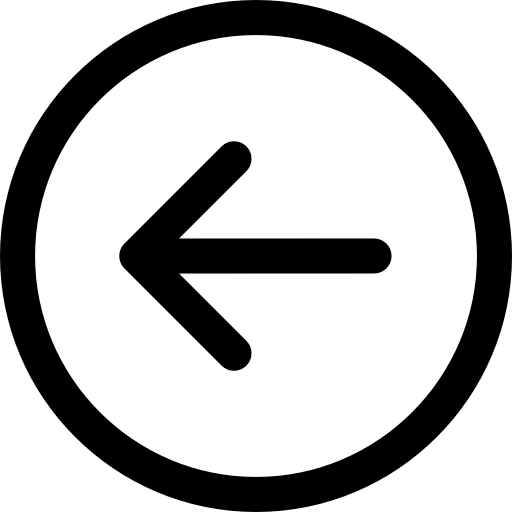``````#include<stdio.h>
#include<math.h>
#include<conio.h>
void main ()
{
int choice,i1,k1,j1,i2,j2,k2,i,j,k,f;
clrscr ();
do
{
printf("\nEnter the choice.\n1-To find AXB of vectors.\n2-To find A.B of vectors.\n0-To Exit\n");
scanf("%d",&choice);
switch(choice)
{
case 1:
printf("You have chosen to find AXB of vectors.So enter the value of i.j.k respectively of vector A.\n");
scanf("%d%d%d",&i1,&j1,&k1);
printf("\nEnter the value of i,j,k respectively of the vector B.\n");
scanf("%d%d%d",&i2,&j2,&k2);
i=+((k2)*(j1)+(j2)*(k1));
j=-((k2)*(i1)+(i2)*(k1));
k=+((j2)*(i1)+(i2)*(j1));
printf("The value of AXB of vector is %di^,%dj^,%dk^.",i,j,k);
break;

case 2:
printf("\nYou have chosen to find A.B of vectors.So enter the value of i,j,k of the vector A.");
scanf("%d%d%d",&i1,&j1,&k1);
printf("\nEnter the value of i,j,k respectively of the vector B.");
scanf("%d%d%d",&i2,&j2,&k2);
i=((i1)*(i2));
j=((j1)*(j2));
k=((k1)*(k2));
f=((i)+(j)+(k));
printf("\nThe value of A.B of the vectors is %d.",f);
break;

default:printf("\nYou have entered a wrong choice.\nPlease enter a valid choice.");
break;
}
}
while(choice!=0);
}
``````